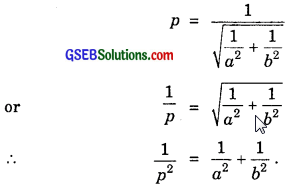# GSEB Solutions Class 11 Maths Chapter 10 Straight Lines Ex 10.3

Gujarat Board GSEB Textbook Solutions Class 11 Maths Chapter 10 Straight Lines Ex 10.3 Textbook Questions and Answers.

## Gujarat Board Textbook Solutions Class 11 Maths Chapter 10 Straight Lines Ex 10.3

Question 1.
Reduce the following equations into slope-intercept form and find their slopes and the intercept:
(i) x + 7y = 0
(ii) 6x + Sy – 5 = 0
(iii) y = 0
Solution:
(i) x + 7y = 0 or y = – $$\frac{1}{7}$$x + 0.
∴ Slope = – $$\frac{1}{7}$$ and y-intercept = 0.

(ii) 6x + 3y – 5 = 0 or 3y = – 6x + 5
y = – 2x + $$\frac{5}{3}$$.
∴ Slope = – 2 and y – intercept = $$\frac{5}{3}$$.

(iii) y = 0 or y = 0x + 0.
∴ Slope = 0, y-intercept = 0.Question 2.
Reduce the following equations into intercepts form and find their intercepts on the axes.
(i) 3x + 2y – 12 = 0
(ii) 4x – 3y = 6
(iii) 3y + 2 = 0
Solution:
(i) 3x + 2y – 12 = 0 or 3x + 2y = 12.
Dividing by 12, we get
$$\frac{x}{4}$$ + $$\frac{y}{6}$$ = 1.
∴ Intercepts on the axes are 4 and 6.

(ii) 4x – 3y = 6 or $$\frac{4}{6}$$x – $$\frac{y}{2}$$ = 1.
or $$\frac{x}{3/2}$$ + $$\frac{y}{-2}$$ = 1.
Intercepts on the axes are $$\frac{3}{2}$$ and – 2.

(iii) 3y + 2 = 0 or 3y = – 2.
or $$\frac{y}{-2/3}$$ = 1 or $$\frac{x}{0}$$ + $$\frac{y}{-2/3}$$ = 1.
Intercepts on the axes are 0 and – $$\frac{2}{3}$$.

Question 3.
Reduce the following equations into the normal form. Find their perpendicular distance from the origin and angle between perpendicular and positive direction of x-axis.
(i) x – $$\sqrt{3}$$y + 8 = 0
(ii) y – 2 = 0
(iii) x – y = 4
Solution:
(i) x – $$\sqrt{3}$$y + 8 = 0 or x – $$\sqrt{3}$$y = – 8.
or – x + $$\sqrt{3}$$y = 8.
Put rcos ω = – 1 and rsin ω = $$\sqrt{3}$$.
Squaring and adding r2 = 1 + 3 = 4, ∴ r = 2.
cos ω = $$\frac{1}{2}$$, sin ω = $$\frac{\sqrt{3}}{2}$$ ∴ ω = 120° = $$\frac{2π}{3}$$.
∴ xcos ω + y sin ω = $$\frac{8}{2}$$ = 4.
∴ p = 4 and ω = $$\frac{2π}{3}$$.

(ii) y – 2 = 0
Comparing it with Ax + By = C, we get
A = 0, B = 1, r = $$\sqrt{0+1^{2}}$$ = 1.
∴ cos ω = 0, sin ω = $$\frac{8}{2}$$ = 4.
∴ y = 2 ⇒ x cos $$\frac{π}{2}$$ + ysin $$\frac{π}{2}$$ = 2
∴ ω = $$\frac{π}{2}$$, p = 2.

(iii) x – y = 4 ………………… (1)
Put r cos ω = 1, r sin ω = – 1.
So, r = $$\sqrt{1^{2}+(-1)^{2}}$$ = $$\sqrt{2}$$
and cos ω = $$\frac{1}{\sqrt{2}}$$, sin ω = $$\frac{1}{\sqrt{2}}$$.
⇒ ω = 360° – 45° = 315°.
Dividing (1) by $$\sqrt{2}$$, we get
$$\frac{1}{\sqrt{2}}$$x + (- $$\frac{1}{\sqrt{2}}$$y) = $$\frac{y}{\sqrt{2}}$$ = 2$$\sqrt{2}$$.
∴ Normal form of the given line is
xcos 315° + ysin 315° = 2$$\sqrt{2}$$.
∴ ω = 315°, p = 2$$\sqrt{2}$$.Question 4.
Find the distance of the point (- 1, 1) from the line 12(x + 6) = 5(y – 2).
Solution:
The given line is
12(x + 6) = 5(y – 2)
or 12x + 72 = 5y – 10
or 12x – 5y + 72 + 10 = 0
or 12x – 5y + 82 = 0.
∴ The perpendicular distance from (x1, y1) to the line ax + by + c = 0 is $$\frac{\left(a x_{1}+b y_{1}+c\right)}{\sqrt{a^{2}+b^{2}}}$$.
The point (x1, y1) is (- 1, 1).
∴ Perpendicular distances from (- 1, 1) to the line 12x – 5y + 82 = 0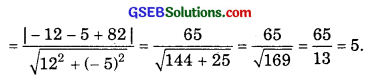Question 5.
Find the points on the x-axis, whose distances from $$\frac{x}{3}$$ + $$\frac{y}{4}$$ = 1 are 4 units.
Solution:
The given line is $$\frac{x}{3}$$ + $$\frac{y}{4}$$ = 1.
Multiplying by 12, we get
4x + 3y = 12 or 4x + 3y – 12 = 0.
Any point on the x-axis is (x, 0).
∴ Perpendicular distance from (x, 0) to the given line
= $$\frac{|4 x-12|}{\sqrt{4^{2}+3^{2}}}$$ = 4.
or $$\frac{|4 x-12|}{\sqrt{25}}$$ = 4 or |4x – 12| = 5 × 4 = 20.
Taking (+ve) sign, 4x – 12 = 20 or 4x = 20 + 12 = 32
⇒ x = $$\frac{32}{4}$$ = 8.
Taking (- ve) sign, 4x – 12 = – 20.
⇒ 4x = – 20 + 12 = – 8
∴ x = – 2.
∴ Required points on the x-axis are (8, 0) and (- 2, 0).Question 6.
Find the distance between the parallel lines:
(i) 15x + 8y – 34 = 0 and 15x + 8y + 31 = 0.
(ii) l(x + y) + p = 0 and lx + ly – r = 0.
Solution:
One of the lines is
15x + 8y – 34 = 0.
Putting y = 0, 15x = 34 or x = $$\frac{34}{15}$$.
∴ The point ($$\frac{34}{15}$$, 0) lies on 15x + 8y – 34 = 0.
∴ Perpendicular distance from ($$\frac{34}{15}$$, 0) to 15x + 8y + 31 = 0 is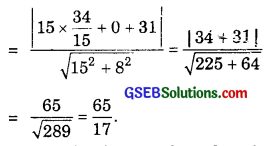Alternative method:
Perpendicular distance from the origin of these lines are p1 = $$\frac{-34}{\sqrt{15^{2}+8^{2}}}$$ = $$\frac{- 34}{17}$$ and p2 = $$\frac{31}{17}$$.
∴ Perpendicular distance between the given parallel lines = |p2 – p1|
= |$$\frac{-34}{17}$$ – $$\frac{31}{17}$$| = |$$\frac{-65}{17}$$| = $$\frac{65}{17}$$.

(ii) The parallel lines are
l(x + y) + p = 0, lx + ly – r = 0.
Perpendicular distances from origin are∴ Perpendicular distance between the given parallel lines are
|$$\frac{p}{\sqrt{2l}}$$ – $$\frac{- r}{\sqrt{2l}}$$| = $$\frac{p+r}{\sqrt{2l}}$$.

Question 7.
Find the equation of a line parallel to the line 3x – 4y + 2 = 0 and passing through the point (- 2, 3).
Solution:
The given line is 3x – 4y + 2 = 0.
∴ Slope of the line = $$\frac{3}{4}$$.
∴ Slope of a parallel line = $$\frac{3}{4}$$.
∴ The line passing through (- 2, 3) and parallel to 3x – 4y + 2 = 0 is
y – 3 = $$\frac{3}{4}$$(x + 2).
or 4y – 12 = 3x + 6
or 3x – 4y + 12 + 6 = 0 or 3x – 4y + 18 = 0.

Alternative Method.
Any line parallel to 3x – 4y + 2 = 0 is 3x – 4y = k.
[Note that the coeffs. of x and y are the same for parallel lines]
The line passes through (- 2, 3).
So, 3(- 2) – 4(3) = k
or k = – 6 – 12 = – 18
∴ Equation of the required line is 3x – 4y = – 18
or 3x – 4y + 18 = 0.Question 8.
Find the equation of the line perpendicular to the line x – 7y + 5 = 0 and having x-intercept 3.
Solution:
The line having the x-intercept 3, passes through the point (3, 0).
The given line is x – 7y + 5 = 0.
∴ Slope of it = $$\frac{1}{7}$$.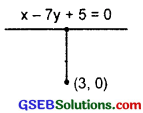∴ Slope of perpendicular line = – 7.
∴ The line passing through (3, 0) and slope – 7 is
y – 0 = – 7(x – 3)
or y = – 7x + 21
∴ 7x + y – 21.

Alternative Method.
Any line perpendicular to ax + by + c = 0 is bx – ay + k – 0.
Note that here coefficients of x and y are interchanged and sign between them also changes from + to – and – to + in between.
∴ The line perpendicular to x – 7y + 5 = 0 is
7x + y = k.
Putting x = 3, y = 0 in it, we get 21 = k.
∴ Equation of the required line is
7x + y = 21.

Question 9.
Find the angles between the lines $$\sqrt{3x}$$ + y = 1 and z + $$\sqrt{3}$$y = 1.
Solution:
The first line is $$\sqrt{3}$$x + y = 1 or y = – $$\sqrt{3x}$$ + 1.
∴ The slope m1 = – $$\sqrt{3}$$
∴ m1 = – $$\sqrt{3}$$.
The other line is x + $$\sqrt{3y}$$ = 1.
or y = – $$\frac{1}{\sqrt{3}}$$x + $$\frac{1}{\sqrt{3}}$$.
∴ The slope m2 of the line x + $$\sqrt{3y}$$ = 1 is – $$\frac{1}{\sqrt{3}}$$.
∴ m2 = – $$\frac{1}{\sqrt{3}}$$.
If θ is the angle between two lines, then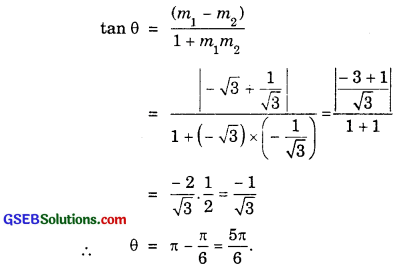So, acute angle between the lines is $$\frac{π}{6}$$.Question 10.
The line through the points (h, 3) and (4, 1) intersects the line 7x – 9y – 19 = 0 at right angles. Find the value of h?
Solution: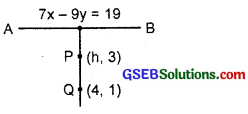Slope of the line PQ passing through P(h, 3) and Q(4, 1).
= $$\frac{3-1}{h-4}$$ = $$\frac{2}{h-4}$$.
Slope of the line AB, i.e; 7x – 9y – 19 = 0 is $$\frac{7}{9}$$.
The lines AB and PQ are perpendicular to each other.
∴ m1m2 = – 1 or $$\frac{2}{h-4}$$ × $$\frac{7}{9}$$ = – 1.
∴ 14 = – 9(h – 4) = – 9h + 36
∴ 9h = 36 – 14 = 22.
∴ h = $$\frac{22}{9}$$.

Question 11.
Prove that the line through the point (x1, y1) and parallel to the line Ax + By + C = 0 is
A(x – x1) + B(y – y1) = 0.
Proof:
The given line is Ax + By + C = 0of y = – $$\frac{A}{B}$$x – $$\frac{C}{B}$$
∴ Slope of the parallel line = – $$\frac{A}{B}$$
The parallel line passes through (x1, y1) is
y – y1 = – $$\frac{A}{B}$$(x – x1)
or B(y – y1) = – A(x – x1)
or A(x – x1) + B(y – y1) = 0.
Alternatively. The line parallel to Ax + By + C = 0 is
Ax + By + k = 0 ………………. (1)
It passes through (x1y1)
∴ Ax1 + By1 + k = 0 ……………… (2)
Subtracting (2) from (1), we get
A(x – x1) + B(y – y1) = 0.Question 12.
Two lines passing through the point (2, 3) intersects each other at an angle of 60°. If slope of one line is 2, find the equation of the other line.
Solution:
Let m be the slope of the other line.
Slope of one line = 2.
Angle between them = 60°.Equation of the line passing through P(2, 3) with slope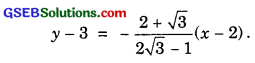or (1 + 2$$\sqrt{3}$$)y – 3(1 + 2$$\sqrt{3}$$) = (2 – $$\sqrt{3}$$x – 2(2 – $$\sqrt{3}$$).
or (1 + 2$$\sqrt{3}$$)y – 3 – 6$$\sqrt{3}$$ = (2 – $$\sqrt{3}$$)x – 4 + 2$$\sqrt{3}$$
∴ (2 – $$\sqrt{3}$$)x – (1 + 2$$\sqrt{3}$$)y – 4 + 2$$\sqrt{3}$$ + 3 + 6$$\sqrt{3}$$ = 0.
or (2 – $$\sqrt{3}$$)x – (1 + 2$$\sqrt{3}$$)y – 1 + 8$$\sqrt{3}$$ = 0.
∴ Required lines are
($$\sqrt{3}$$ + 2)x + (2$$\sqrt{3}$$ – 1)y – 8$$\sqrt{3}$$ – 1 = 0.
and (2 – $$\sqrt{3}$$)x – (1 + 2$$\sqrt{3}$$)y + 8$$\sqrt{3}$$ – 1 = 0.Question 13.
Find the equation of the right bisector of the line segment joining the points (3, 4) and (- 1, 2).
Solution:
Slope of the line joining the points A(- 1, 2) and B(3, 4)
= $$\frac{4-2}{3+1}$$ = $$\frac{2}{4}$$ = $$\frac{1}{2}$$.Right bisector PQ is perpendicular to AB.
∴ Slope of PQ = – 2.
Middle point of AB is ($$\frac{-1+3}{2}$$, $$\frac{2+4}{2}$$), i.e; (1, 3).
Right bisector passes through M(1, 3).
∴ Equation of right bisector PQ is
y – 3 = – 2(x – 1) = – 2x + 2.
or 2x + y – 3 – 2 = 0
or 2x + y – 5 = 0.

Question 14.
Find the co-ordinates of the foot of the perpendicular from a point (- 1, 3) to the line 3ax – 4y – 16 = 0.
Solution:
The equation of the given line is 3x – Ay – 16 = 0.
The equation of a line perpendicular to the given line is
4x + 3y + k = 0, where k is a constant.
If this line passes through the point (- 1, 3), then
– 4 + 9 + k = 0 ⇒ k = – 5.
∴ The equation of the line passing through the point (- 1, 3) and perpendicular to the given line is
4x + 3y – 5 = 0.
∴ The required foot of the perpendicular is the point of intersection of the lines
3x – 4y – 16 = 0 …………… (1)
and 4x + 3y – 5 = 0 ……………….. (2)
Solving (1) and (2), by cross-multiplication, we have: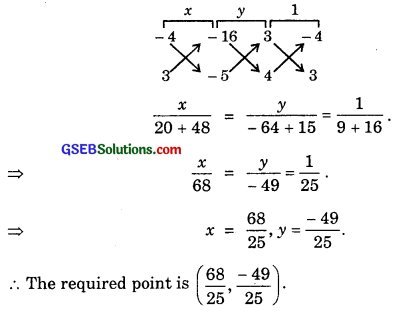Question 15.
The perpendicular from the origin to the line y = mx + c meets it at the point (- 1, 2). Find the value of m and c.
Solution:
Let the perpendicular OM is drawn from the origin to AB. M is the foot of the perpendicular.
Slope of OM = $$\frac{2-0}{-1-0}$$ = $$\frac{2}{-1}$$.
Slope of AB = m
OM ⊥ AB.
∴ m × (- 2) = – 1
∴ m = $$\frac{1}{2}$$.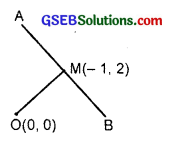M(- 1, 2) lies on y = mx + c or y = $$\frac{1}{2}$$x + c.
∴ 2 = $$\frac{1}{2}$$ × (- 1) + c, c = 2 + $$\frac{1}{2}$$ = $$\frac{5}{2}$$.
∴ m = $$\frac{1}{2}$$ and c = $$\frac{5}{2}$$.

Question 16.
If p and q are the lengths of perpendiculars from the origin to the lines xcos θ – ysin θ = kcos 2θ and x sec θ + y cosec θ = k respectively, prove that p2 + 4q2 = k2.
Solution:
The perpendicular distance from the origin to the line xcos θ – ysin θ = kcos 2θ ………………. (1)∴ The perpendicular distance q from the origin to the line (2) isQuestion 17.
In the triangle ABC with vertices A(2, 3), B(4, – 1) and C(1, 2), find the equation and length of altitude from the vertex A.
Solution:
The vertices of ∆ ABC are A(2, 3), B(4, – 1) and C(1, 2) and AM is the altitude.
Slope of BC = $$\frac{2+1}{1-4}$$ = $$\frac{3}{-3}$$ = – 1.
∴ Slope of altitude AM = 1. [∵ m1m2 = – 1]Now, altitude passes through A(2, 3) and has the slope 1.
∴ Equation of AM is
y – 3 = 1(x – 2).
or x – y + 3 – 2 = 0
or y – x = 1.
Equation of BC passing through B(4, – 1) and C(1, 2) is
y + 1 = – (x – 4).
or x + y = 3
or x + y – 3 = 0.
∴ Length of altitude = AM
= Perpendicular distance from A(2, 3) to BC
= $$\frac{2+3-3}{\sqrt{1^{2}+1^{2}}}=\frac{2}{\sqrt{2}}=\sqrt{2}$$
∴ Equation of altitude is y – x = 1 and length of altitude = $$\sqrt{2}$$.Question 18.
If p is the length of perpendicular from the origin to the line whose intercepts on the axes are a and b, then show that $$\frac{1}{p^{2}}$$ = $$\frac{1}{a^{2}}$$ + $$\frac{1}{b^{2}}$$.
Solution:
Equation of the line which makes intercepts a and b on the axes is
$$\frac{x}{a}$$ + $$\frac{y}{b}$$ = 1.∴ The perpendicular distance p from the origin is given by